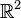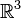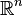# Euclidean space facts for kids

Kids Encyclopedia Facts

Euclidean space is the space Euclidean geometry uses. In essence, it is described in Euclid's Elements. The Euclidean plane ($\mathbb{R}^2$) and three-dimensional space ($\mathbb{R}^3$) are part of Euclidean space, which can be generalized to any dimension n (in which case one writes$\mathbb{R}^n$).

Euclidian space is sometimes called Cartesian space. A few other spaces and mathematical objects that are similar were added later. Euclid based his work Elements on certain axioms. Euclidean space can be described using such axioms.

## Images for kidsEuclidean space Facts for Kids. Kiddle Encyclopedia.